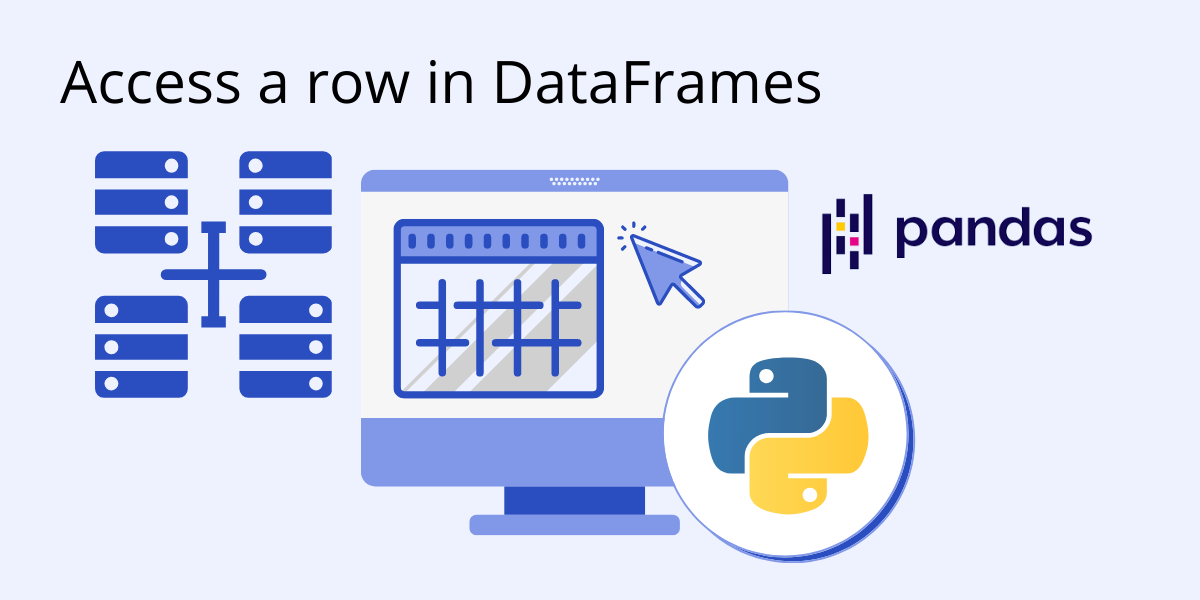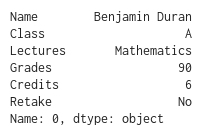# How to Access a Row in a DataFrameBefore we start: This Python tutorial is a part of our series of Python Package tutorials. The steps explained ahead are related to the sample project introduced here.
You can use the loc and iloc functions to access rows in a Pandas DataFrame. Let’s see how.

In our DataFrame examples, we’ve been using a Grades.CSV file that contains information about students and their grades for each lecture they’ve taken:Now let’s imagine we needed the information for Benjamin’s Mathematics lecture. We could simply access it using the iloc function as follows:

`Benjamin_Math  = Report_Card.iloc`The above function simply returns the information in row 0. This is useful, but since the data is labeled, we can also use the loc function:

```Benjamin_Math =
Report_Card.loc[(Report_Card["Name"]     =="Benjamin Duran") &
(Report_Card["Lectures"] == "Mathematics")]```

In this case, we are using  simple logic to index our DataFrame:

• First, we check for all rows where the Name column is Benjamin Duran
• Within that result, we then look for all rows where the Lectures column is Mathematics.

This will return us a DataFrame matching the result of the iloc example above.

And if we wanted to access Benjamin’s Mathematics grade and store it in a variable, we could simply do the following:

`grade = Benjamin_Math["Grades"]`

Since we only have one row of information, we can simply index the Grades column, which will return us the integer value of the grade.

#### Next steps

Now that you know how to access a row in a DataFrame using Python’s Pandas library, let’s move on to other things you can do with Pandas:

## Python For Data Science

Pre-bundled with the most important packages Data Scientists need, ActivePython is pre-compiled so you and your team don’t have to waste time configuring the open source distribution. You can focus on what’s important–spending more time building algorithms and predictive models against your big data sources, and less time on system configuration.

### Some Popular Python Packages for Data Science/Big Data/Machine LearningYou Get Pre-compiled – with ActivePython

• pandas (data analysis)
• NumPy (multi-dimensional arrays)
• SciPy (algorithms to use with numpy)
• HDF5 (store & manipulate data)
• Matplotlib (data visualization)
• Jupyter (research collaboration)
• PyTables (managing HDF5 datasets)
• HDFS (C/C++ wrapper for Hadoop)
• pymongo (MongoDB driver)
• SQLAlchemy (Python SQL Toolkit)
With deep roots in open source, and as a founding member of the Python Foundation, ActiveState actively contributes to the Python community. We offer the convenience, security and support that your enterprise needs while being compatible with the open source distribution of Python.